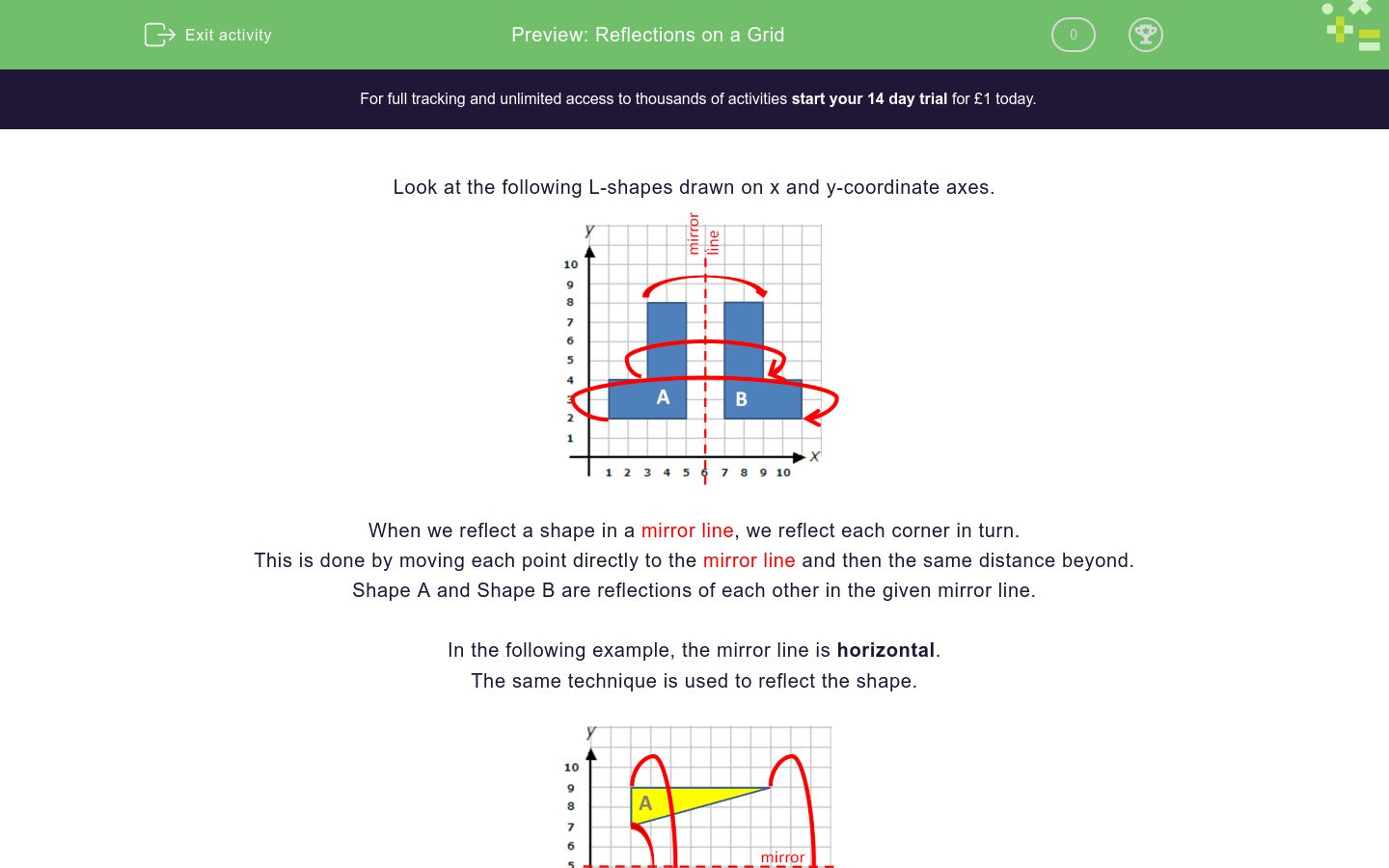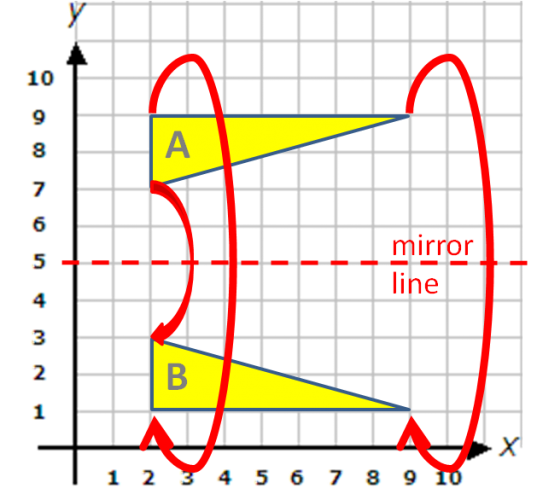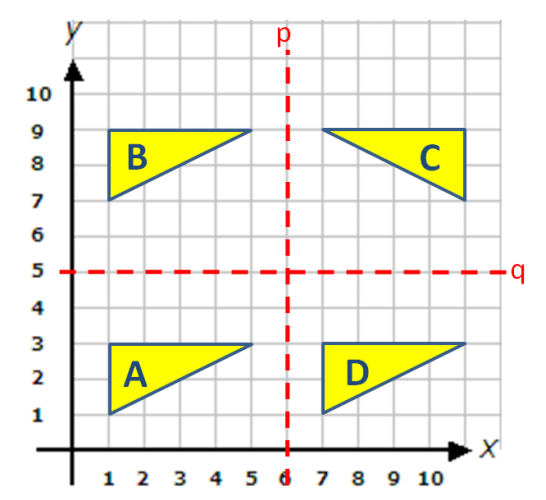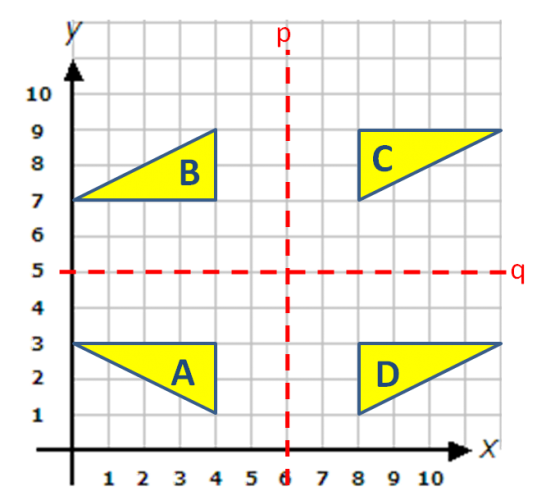# Reflections on a Grid

In this worksheet, students must understand how to reflect a shape in a vertical or horizontal mirror line.Key stage:  KS 2

Curriculum topic:   Geometry: Position and Direction

Curriculum subtopic:   Know, Describe and Present Shapes

Difficulty level:### QUESTION 1 of 10

Look at the following L-shapes drawn on x and y-coordinate axes.When we reflect a shape in a mirror line, we reflect each corner in turn.

This is done by moving each point directly to the mirror line and then the same distance beyond.

Shape A and Shape B are reflections of each other in the given mirror line.

In the following example, the mirror line is horizontal.

The same technique is used to reflect the shape.Look at the shapes on this grid.

Which two shapes are reflections of each other in the line p?A

B

C

D

Look at the shapes on this grid.

Which two shapes are reflections of each other in the line p?A

B

C

D

Look at the shapes on this grid.

Which two shapes are reflections of each other in the line p?A

B

C

D

Look at the shapes on this grid.

Which two shapes are reflections of each other in the line q?A

B

C

D

Look at the shapes on this grid.

Which two shapes are reflections of each other in the line q?A

B

C

D

Look at the shapes on this grid.

Which two shapes are reflections of each other in the line p?A

B

C

D

Look at the shapes on this grid.

Is this statement true or false?

A is a reflection of C in line q.true

false

Look at the shapes on this grid.

Is this statement true or false?

A is a reflection of B in line q.true

false

Look at the shapes on this grid.

Is this statement true or false?

D is a reflection of B in line q.true

false

Look at the shapes on this grid.

Is this statement true or false?

D is a reflection of C in line q.true

false

• Question 1

Look at the shapes on this grid.

Which two shapes are reflections of each other in the line p?A
B
EDDIE SAYS
Line p is a mirror line for the shapes A and B.
C and D are translations of A.
• Question 2

Look at the shapes on this grid.

Which two shapes are reflections of each other in the line p?B
C
EDDIE SAYS
Line p is a mirror line for the shapes B and C.
A and D are translations of B.
• Question 3

Look at the shapes on this grid.

Which two shapes are reflections of each other in the line p?C
D
EDDIE SAYS
Line p is a mirror line for the shapes C and D.
A and B are translations of each other.
• Question 4

Look at the shapes on this grid.

Which two shapes are reflections of each other in the line q?B
D
EDDIE SAYS
Line q is a mirror line for the shapes B and D.
A and C are not reflections because they have turned.
• Question 5

Look at the shapes on this grid.

Which two shapes are reflections of each other in the line q?A
B
EDDIE SAYS
Line q is a mirror line for the shapes A and B.
C and D are translations of each other.
• Question 6

Look at the shapes on this grid.

Which two shapes are reflections of each other in the line p?A
D
EDDIE SAYS
Line p is a mirror line for the shapes A and D.
B and C are not reflections because they have turned.
• Question 7

Look at the shapes on this grid.

Is this statement true or false?

A is a reflection of C in line q.false
EDDIE SAYS
A and C are translations of each other.
• Question 8

Look at the shapes on this grid.

Is this statement true or false?

A is a reflection of B in line q.false
EDDIE SAYS
A and B are reflections of each other but in line p.
• Question 9

Look at the shapes on this grid.

Is this statement true or false?

D is a reflection of B in line q.true
EDDIE SAYS
Line q is a mirror line for shapes B and D.
• Question 10

Look at the shapes on this grid.

Is this statement true or false?

D is a reflection of C in line q.false
EDDIE SAYS
D is a translation of C. It has not flipped over the mirror line.
---- OR ----

Sign up for a £1 trial so you can track and measure your child's progress on this activity.

### What is EdPlace?

We're your National Curriculum aligned online education content provider helping each child succeed in English, maths and science from year 1 to GCSE. With an EdPlace account you’ll be able to track and measure progress, helping each child achieve their best. We build confidence and attainment by personalising each child’s learning at a level that suits them.

Get started